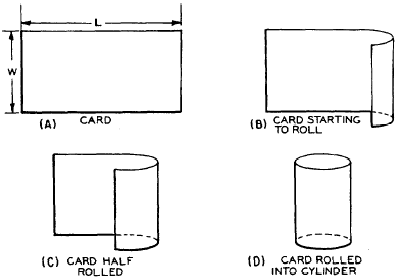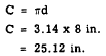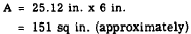Custom SearchSurface Area and Volume The lateral area of a cylinder is the area of its curved surface, excluding the area of its bases. Figure 18-15 illustrates an experimental method of determining the lateral area of a right circular cylinder. The card of length L and width W in figure 18-15 is rolled into a cylinder. The height of the cylinder is W and the circumference is L. The lateral area is the same as the original area of the card, LW. Therefore, the lateral area of the cylinder is found by multiplying its height by the circumference of its base. Written as a formula, this is A = Ch EXAMPLE: Find the lateral area of a right circular cylinder whose base has a radius of 4 inches and whose height is 6 inches.Figure 18-15.-Lateral area of a cylinder. SOLUTION: The circumference of the base isTherefore,The formula for the volume of a cylinder is obtained by the same reasoning process that was used for prisms. The cylinder is considered to be composed of many circular wafers, or disks, each one unit thick. The area of each disk, multiplied by the number of disks, is the volume of the cylinder. With V representing volume, A drepresenting the area of each disk, and n representing the number of disks, the formula is as follows: V = Adn Since the number of disks is the same as the height of the cylinder, the formula for the volume of a cylinder is normally written V = Bh In this formula, B is the area of the base and h is the height of the cylinder. EXAMPLE: Determine the volume of a circular cylinder with a base of radius 5 inches and a height of 14 inches. SOLUTION:Practice problems:  1. Determine the lateral area of a right circular cylinder with a base of diameter 7 inches and a height of 4 inches. 2. Determine the volume of the cylinder in problem 1. Answers: 1. 88 sq in.  2. 154 cu in.Integrated Publishing, Inc. - A (SDVOSB) Service Disabled Veteran Owned Small Business